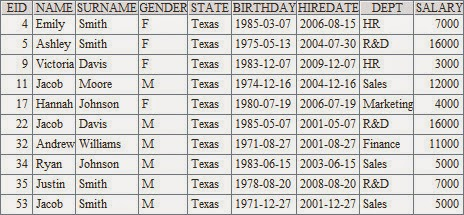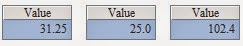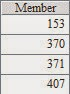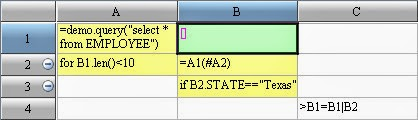## July 30, 2014

### Loop Code for esProc

With esProc, the code for loop is mainly implemented with for statement. The for statement will repeat the code block with for as the main cell. There are different formats of the for statements, as listed below:

1.The for loop
Unconditional loop. The values of the main cell are, in turn, the count for the current loop, which often needs to be exited by break command.
Unconditional loop or endless loop is the simplest structure, for example:

In the above example, A2 executes unconditional loop. According to the loop number in A2, B2 retrieves one employee record every time. The rest of codes save the employee information for Texas in B1. After the information for first 10 Texas employees were retrieved, the loop will jump out with break statement in C6. Alternatively, break C command can also be used to replace break statement to jump out from the loop body with cell C as the main cell.
After execution, B1 contains the following results:Note that when using the unconditional loop, we need to make sure that the break statement can be executed properly, otherwise theprogram will never end at the time of execution.

2.for A
Loop the member of the sequence A, the main cell value will in turn be the current member of A.

In esProc, the most commonly used loop is to loop on every member in a sequence. For example:In this example, all Texas employees are selected in A2. The loop is executed in A3 for each Texas employee to compute their age, and then store the oldest age in B1. When the loop is complete, we can get the maximum age for all Texas employees from B1.
For relatively simple loop statement, sometimes we can use the loop function for sequence to achieve the same result. For example:3.for n
Loop n times;with the main cell value as the current loop count in turn.

In addition to loop on all members in a sequence, loop for specific times is also very common.
For example, suppose we have a piece of paper which is 1000mm * 800mm in size and 0.1mm in thickness. If we fold it ten times, what will be the final length, width and thickness?In the above example, the folding is looped ten times. After each folding the length will be reduced by half, and the thickness will be doubled. If after the folding the width of the paper is greater than the length, the length and width will be switched. After execution, A1, B1 and C1 contains the length, width and thickness of the paper as following:The for n statement can also be seen as a simple form of for to (1, n) . This means "loop from 1 to n".

4.for a, b, s
Loop from a to b, at the step of s. This means "to loop on each member of array to(a, b).step (s)". If s is absent the default value will be 1.
Sometimes the loop does not start from 1, and the step value is not 1. We can then use for a, b, s loop. For example:For any n digits number, if the total ofthe n-th power of each digit equals its own, the number can be called daffodils number. The following example is used to identify all three digits daffodils number:In the loop codesin A2, the loop is excecuted on all 3 digits numbers. After the execution of program, we can see the result in A1:5.for x
Loop when x is true. The value in main cell is the computed value for x.

By specifying the loop condition, we can control when to end the loop. For example, the issue in section 1 can also be solved with for statementwith specific loop conditions:In the main cell of for loop, the cell value is either true or false, which is the result we got after computing the loop condition every time. At this point we can not use the value from main cell as the count of loop. Therefore to retrieve employee records in B2, we use #C to get the current number of loops. In #C, C is the main cell of loop. After the code is executed, the result is the same as in section 1.

6.for cs,n:x
Loop cursor, and retrieve n records each time, or retrieve records until x has changed. Return table sequence. Close after the reading.
When retrieve the data with cursor, we can use for statement to loop the data in cursor.7.Nested loop
In the body of the loop, we can also use loop statement. This is what we call nested loops, or multi-layer loops. For example:In the example, A1 stores the prime number sequencewe found; A2 loops the integer from 2 to 1000. The prime number sequence is looped in B2. If the number in A2 is divisible by certain prime number, it will be replaced by the next number in A2 with the command of next A2. If the number in A2 is not divisible by all integer number in current prime number list, we then find a new prime number. It will be added to the sequence in A1. Among them, the next C command in D2 specifies to skip the rest part of the loop body and enter the next round of loop. After execution, the sequence for prime numbers within 1000 are as following in A1:When using the next statement, we don't have to specify the main cell C, to skip the deepest level of the loop where the next statement is located.
As with the following one hundred chicken puzzle: each rooster is worth of 5 dollars, and each hen 3 dollars. Three chicks is worth of one dollar. If we can buy 100 checken with 100 dollars, how many roosters, hens and chicks do we buy actually?We can resolve the issue in the following way:In the codes, A2 loops on the total number of rooster. We need to judge in B2: if the total price of the rooster is more than 100, there must be too many roosters. We can use break command in C2 to end the loop. In B3 we continue to loop with the possible number of hens. If the current total price of chicken is more than 100 in D4,we know that there are too many hens. We can use next A2 to add the number of roosters and try again. increasing the total number of cocks and try again. In D5, the current total price of chicken is still less than 100. We can continue to increase the total number of hens and try. The next statement here can skip from the deepest level of loop, which has B3 as the main cell.